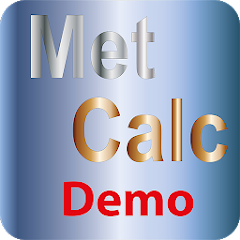# MetCalc-Demo Calculations for

10+Everyone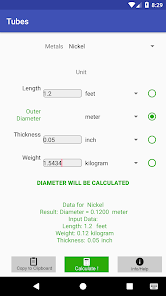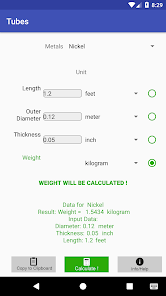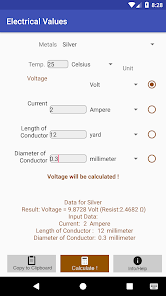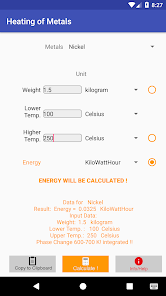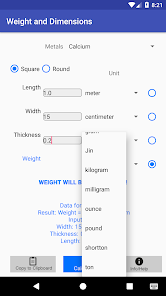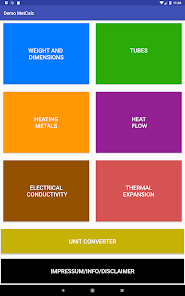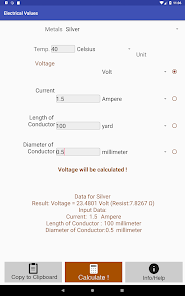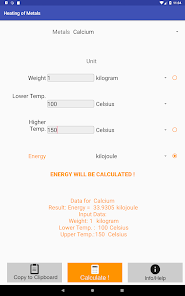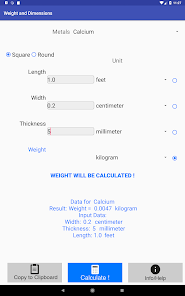MetCalc DEmo is an app which calculates metal values ​​for Nickel, Silver and Calcium. It is a test version for the MetCalc app which calculates values ​​for 27 metals from aluminum to zirconium.
As a basis for the calculation of the specific properties like density, specific heat capacities (for several metals even as a dependence from temperature, including melting energy), thermal conductivity, electrical conductivity (for several metals ie the dependence of temperature). To proof the correctness of the specific values ​​for the user, the source of the values ​​and the uses specific values ​​can be shown.
The results and the input values ​​can be given in a lot of units (eg energy in joule, kilojoules, calories, kilocalories, BTU).
* What is the weight of metal cake or sheet with a certain width, length and thickness?
How long is a coil of metal rod with a certain weight and a certain diameter?
* How long is a strip of nickel rolled down to a certain thickness at a given weight and width?
* What is the weight of tube with a certain thickness, diameter and length?
What is the heat flow through a metal with a certain area, thickness and temperature difference?
* What is the voltage drop in a metal at a certain current, diameter, length and temperature?
* What is the linear expansion of a metal rod of a certain length by heating?

Another feature are the calculations to different targets: As an example you can calculate weight by given length, width and thickness. But you can calculate length by given weight, width and thickness.
The results may be copied to clipboard in a way that they can be used in a spreadsheet program.
The app is suitable for all engineers, scientists, lecturers and craftsmen working with metals.
There are often different specific values ​​in the literature and some calculations are there for the correctness of the calculated values.
Updated on
Nov 20, 2018

## Data safety